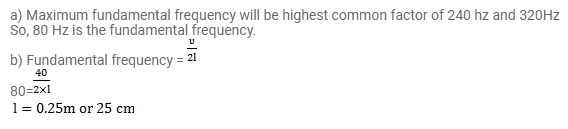# A wire, fixed at both ends is seen to vibrate at a resonant frequencyQuestion:

A wire, fixed at both ends is seen to vibrate at a resonant frequency of $240 \mathrm{~Hz}$ and also at $300 \mathrm{~Hz}$ a. What could be the maximum value of the fundamental frequency?

b. If transverse waves can travel on this string at a speed $0 f 40 \mathrm{~m} / \mathrm{s}$, what is its length?

Solution: